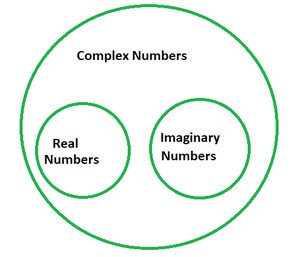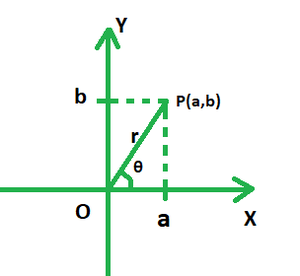# Find a complex number a + bi such that a2 + b2 is irrational

• Last Updated : 30 Dec, 2021

Real and imaginary numbers combine to form complex numbers. The imaginary component, I (iota), indicates a square root of -1. The imaginary portion of a complex number is i. a + ib is a typical representation of complex numbers in their rectangular or standard form. 420 + 69i, for example, is a complex number in which 420 represents the real part and 69 represents the imaginary part.### Modulus

When a complex number is presented on a graph, its real part is plotted on the x-axis and the imaginary part on the y- axis. Say if the number were to be represented by point P in the figure given below, triangles OPA and OPB would be both right-angled. Clearly, in the right triangle POA, PO is the hypotenuse; Oa is the base and Pa is the perpendicular. Using Pythagoras Theorem, we have:

OP2 = OA2 + PA2

OP =The absolute value of a complex number is regarded as its modulus. It is the square root of the sum of squares of its real and imaginary parts. In the above case, OP is the modulus of the complex number of the form z = a + ib, and is denoted by r.### Find a complex number a + bi such that a2 + b2 is irrational.

Solution:

An irrational number is the one which can be expressed in the form of a/b, where b ≠ 0, like √2, √3, etc.

Say we are given the complex number 1 +.

Comparing this with the form a + ib, we have:

a = 1, b =Now, a2 + b2= 1 + 42/3

Clearly, 42/3 cannot be expressed in the form of a/b. Hence it is an irrational number.

### Similar Problems

Question 1. Prove that the square of the modulus ofis irrational.

Solution:

Comparing this with the form a + ib, we have:

a = 0, b =Now, a2 + b2= 22/3

Clearly, 22/3 cannot be expressed in the form of a/b. Hence it is an irrational number.

Question 2. Prove that the square of the modulus ofis irrational.

Solution:

Comparing this with the form a + ib, we have:

a = 0, b =Now, a2 + b2= 22/5

Clearly, 22/5 cannot be expressed in the form of a/b. Hence it is an irrational number.

Question 3. Prove that the square of the modulus of 3 +is irrational.

Solution:

Comparing this with the form a + ib, we have:

a = 3, b =Now, a2 + b2= 9 + 22/3

Clearly, 22/3 cannot be expressed in the form of a/b. Hence it is an irrational number.

Question 4. Prove that the square of the modulus ofis rational.

Solution:

Comparing this with the form a + ib, we have:

a = 4, b == 2

Now, a2 + b2 = 42 + 22

= 20

Clearly, 20 can be expressed in the form of a/b. Hence it is a rational number.

Question 5. Prove that the square of the modulus of 10 +is irrational.

Solution:

Comparing this with the form a + ib, we have:

a = 10, b =Now, a2 + b2= 100 + 22/3

Clearly, 22/3 cannot be expressed in the form of a/b. Hence it is an irrational number.

My Personal Notes arrow_drop_up
Recommended Articles
Page :